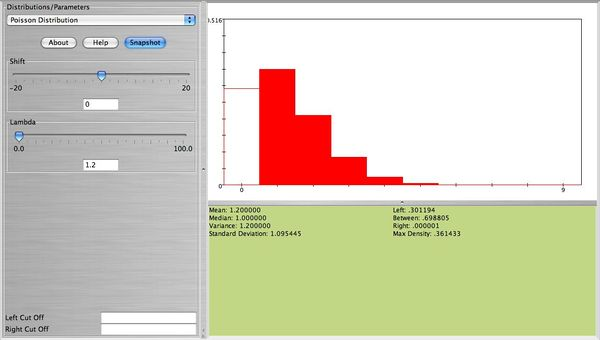# SOCR EduMaterials Activities Explore Distributions

## Summary

This is a SOCR activity to explore the relationships among some of the commonly used probability distributions. This is a complementary SOCR activity to the SOCR Distributions Activity.

## Set-up

You can access the SOCR Distribution applets for these activities by going to this URL page (use a Java-enabled browser) http://www.socr.ucla.edu/htmls/SOCR_Distributions.html.

## Geometric probability distribution

Suppose we roll two dice until a sum of 10 is obtained. What is the probability that the first sum of 10 will occur after the 5th trial? The answer to this question is $$P(X>5)=(1-\frac{3}{36})^5=0.6472.$$. This is equivalent to the event that no sum of 10 is observed on the first 5 trials (5 failures). Now, using SOCR we can obtain this probability easily by entering in the SOCR geometric distribution applet $$p=\frac{3}{36}=0.0833$$ and in the Right Cut-Off box 5. We can find the desire probability on the right corner of the applet. The figure below clearly displays this probability.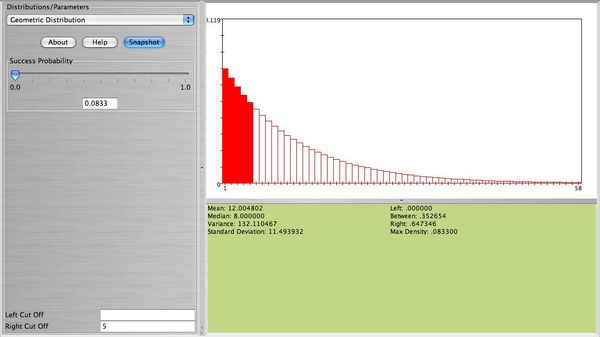## Binomial approximation to hypergeometric

An urn contains 50 marbles (35 green and 15 white). Fifteen marbles are selected without replacement. Find the probability that exactly 10 out of the 15 selected are green marbles. The answer to this question can be found using the formula: $P(X=10)=\frac{{35 \choose 10}{15 \choose 5}}{50 \choose 15}=0.2449$. Using SOCR simply enter population size 50, sample size 15, and number of good objects 35, to get the figure below.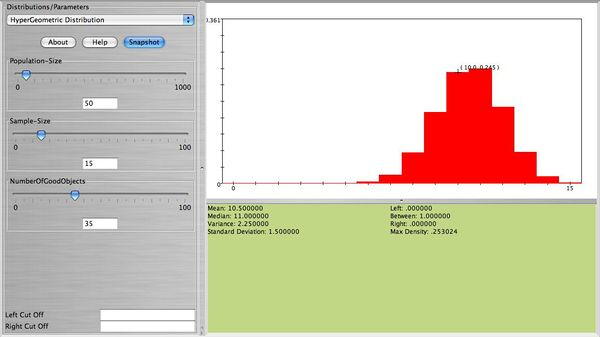Now, select without replacement only 2 marbles. Compute the exact probability that 1 green marble is obtained. This is equal to $P(X=1)=\frac{{35 \choose 1}{15 \choose 1}}{50 \choose 2}=0.4286$. This is also shown on the figure below.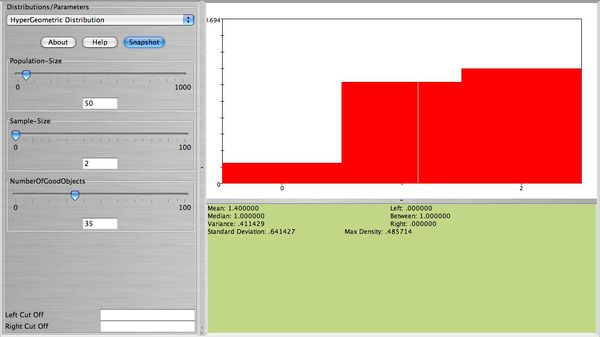We will approximate the probability of obtaining 1 green marble using Binomial as follows. Select the SOCR Binomial distribution and choose number of trials 2 and probablity of success $$p=\frac{35}{50}=0.7$$. Compare the figure below with the figure above. They are almost the same! Why? Using the Binomial formula we can compute the approximate probability of observing 1 green marble as $$P(X=1)={2 \choose 1}0.70^10.30^1=0.42$$ (very close to the exact probability, 0.4286).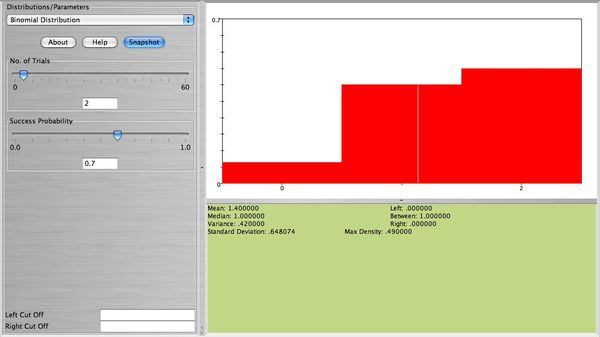## Normal approximation to Binomial

Graph and comment on the shape of binomial with $$n=20, p=0.1$$ and $$n=20, p=0.9$$. Now, keep $$n=20$$ but change $$p=0.45$$. What do you observe now? How about when $$n=80, p=0.1$$. See the four figures below.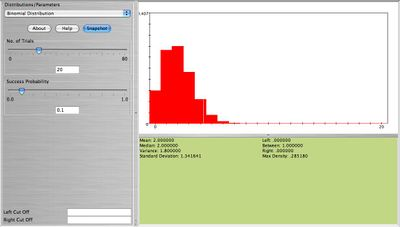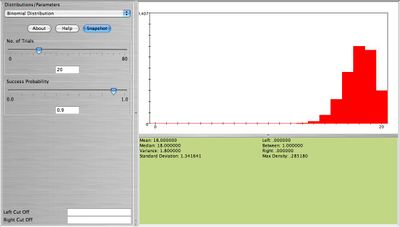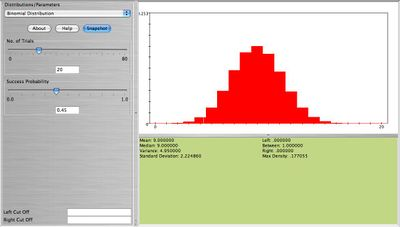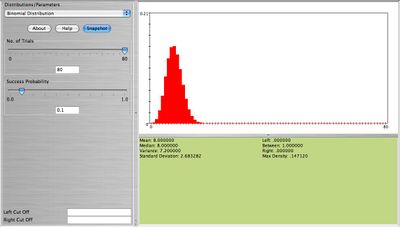What is your conclusion on the shape of the Binomial distribution in relation to its parameters $$n, p$$? Clearly when $$n$$ is large and $$p$$ small or large the result is a bell-shaped distribution. When $$n$$ is small (10-20) we still get approximately a bell-shaped distribution as long as $$p \approx 0.5$$. Because of this feature of the Binomial distribution we can approximate Binomial distributions using the normal distribution when the above requirements hold. Here is one example: Eighty cards are drawn with replacement from the standard 52-card deck. Find the exact probability that at least 8 aces are obtained. This can be computed using the formula $$P(X \ge 8)=\sum_{x=8}^{80}(\frac{4}{52})^x (\frac{48}{52})^{80-x}=0.2725$$.

Much easier we can use SOCR to compute this probability (see figure below).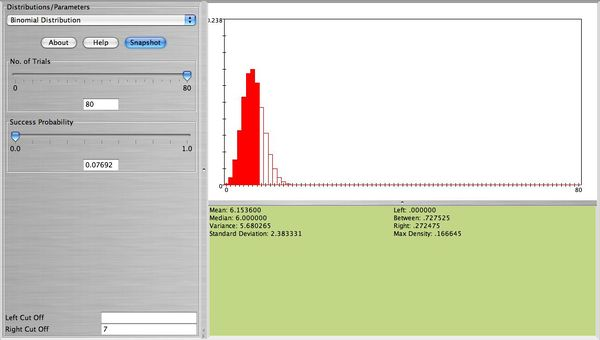But we can also approximate this probability using the normal distribution. We will need the mean and the standard deviation of this normal distribution. These are $$\mu=np=80\frac{4}{52}=6.154$$ and $$\sigma=\sqrt{80 \frac{4}{52}\frac{48}{52}}=2.383.$$ Of course this can be obtained directly from the SOCR Binomial applet. Now, all you need to do is to select the SOCR normal distribution applet and enter for the mean 6.154, and for the standard deviation 2.383. To obtain the desire probability in the right cut-off box enter 7.5 (using the continuity correction for better approximation). The approximate probability is $$P(X \ge 8) \approx 0.2861$$ (see figure below).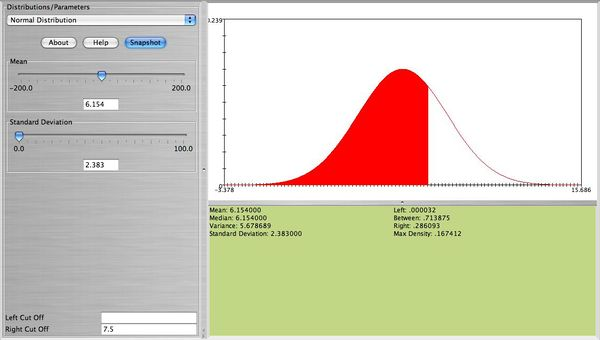## Normal approximation to Poisson

The Poisson distribution with parameter $$\lambda$$ can be approximated with normal when $$\lambda$$ is large. Here is one example. Suppose cars arrive at a parking lot at a rate of 50 per hour. Let’s assume that the process is a Poisson random variable with $$\lambda=50$$. Compute the probability that in the next hour the number of cars that arrive at this parking lot will be between 54 and 62. We can compute this as follows$P(54 \le X \le 62) = \sum_{x=54}^{62} \frac{50^x e^{-50}}{x!}=0.2617.$ The figure below from SOCR shows this probability.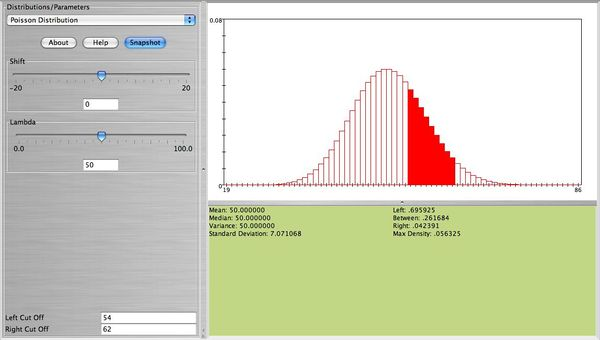• Note: We observe that this distribution is bell-shaped. We can use the normal distribution to approximate this probability. Using $$N(\mu=50, \sigma=\sqrt{50}=7.071)$$, together with the continuity correction for better approximation we obtain $$P(54 \le X \le 62)=0.2718$$, which is close to the exact that was found earlier. The figure below shows this probability.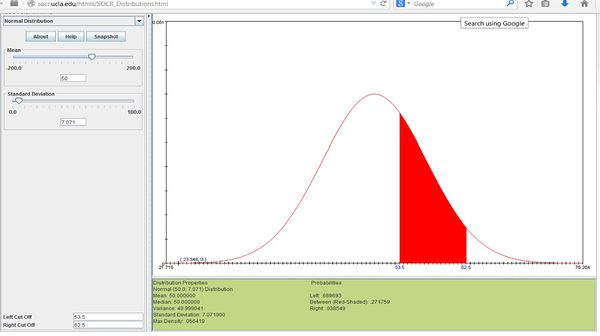## Poisson approximation to Binomial

The Binomial distribution can be approximated well by Poisson when $$n$$ is large and $$p$$ is small with $$np < 7$$. This is true because $$\lim_{n \rightarrow \infty} {n \choose x} p^x(1-p)^{n-x}=\frac{\lambda^x e^{-\lambda}}{x!}$$, where $$\lambda=np$$. Here is an example. Suppose $$2\%$$ of a certain population have Type AB blood. Suppose 60 people from this population are randomly selected. The number of people $$X$$ among the 60 that have Type AB blood follows the Binomial distribution with $$n=60, p=0.02$$. The figure below represents the distribution of $$X$$. This figure also shows $$P(X=0)$$.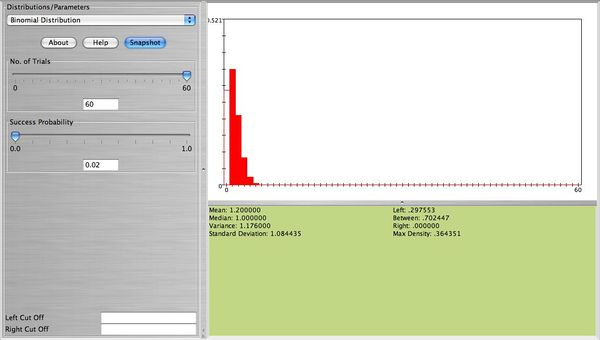• Note: This distribution can be approximated well with Poisson with $$\lambda=np=60(0.02)=1.2$$. The figure below is approximately the same as the figure above (the width of the bars is not important here. The height of each bar represents the probability for each value of $$X$$ which is about the same for both distributions).